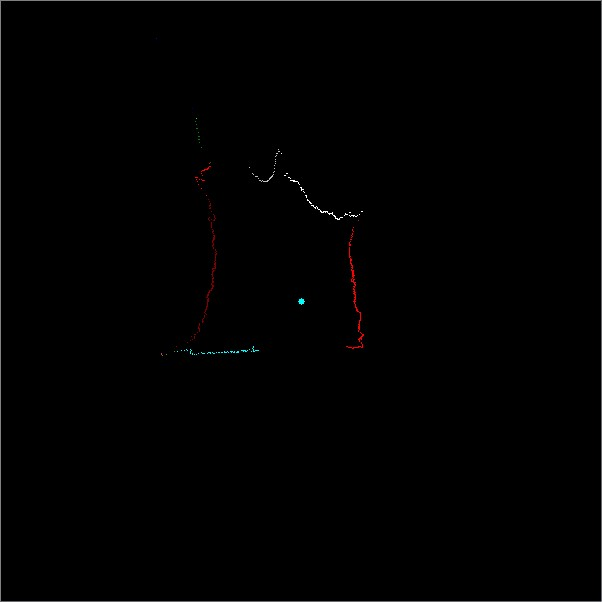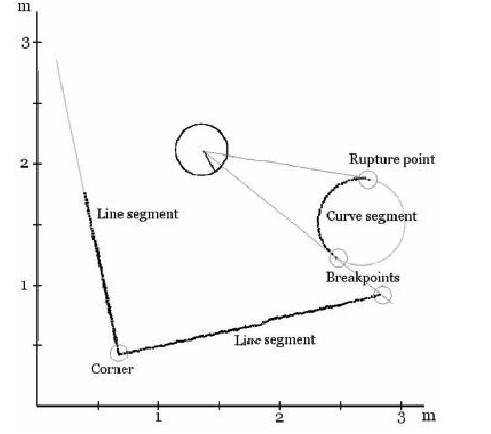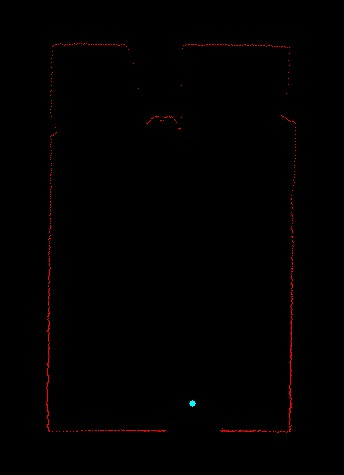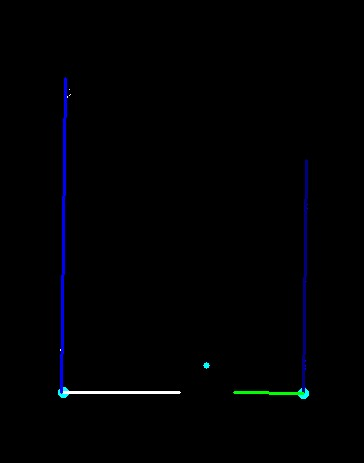# 激光雷达--特征提取

UTM-30LX 有效距离60m 角度分辨率0.25度，，但是一般对于室内应用一般不会超过10m

a、计算相邻两个点之间的距离Dj
b、判断Dj和阈值delta的关系

c、（可选）判断每个区域内的数据点的个数,如果某个区域包含数据点的个数小于等于三个,那么该区域被视为噪声区域,舍弃这些噪声点

//激光雷达区域分割效果，不同的区域用不同的颜色分割（同一种颜色并不代表在同一区域，只是颜色有限，几种颜色在循环使用）1、角点检测

//多边形拟合的方式确定是否存在角点，以及角点的位置

[cpp] view plain
1. // 进行多边形拟合： Points : 轮廓上的点      n -- 轮廓点数目  Eps -- 拟合精度
2. // 返回值： 若该轮廓段需要分段，则返回分段点在该轮廓点列中的索引，否则，返回 0 表示不需要分段
3. // 这里是整个算法计算复杂性最大的一个地方
4. // 为了提高程序运行效率，对点到直线的距离计算进行改进：
5. // 多边形拟合中的直线是由点列中的点决定的
6. // 为了计算点到直线的距离，
7. // 采用坐标系旋转，将直线旋转到x轴方向，这样点到直线的距离即为各个点
8. // 在坐标旋转后的y值的绝对值
9. // 同时，坐标旋转矩阵在该次运算中为定值，只需一次计算，不需要多次的开方或三角计算
10. int OpenRadar::PolyContourFit( int* X, int* Y, int n , float Eps ) // 根据轮廓点，用多边形拟合该轮廓点
11. {
12.     double dis = sqrt((double)(((X - X[n - 1])*(X - X[n - 1])) +
13.                      ((Y - Y[n - 1])* (Y - Y[n - 1]))));
14.     double cosTheta = (X[n- 1] - X) / dis;
15.     double sinTheta = - ( Y[n- 1] - Y )/dis;
16.     double MaxDis = 0;
17.     int i ;
18.     int MaxDisInd = -1;
19.     double dbDis;
20.     for(i = 1 ; i < n - 1 ; i++)
21.     {
22.         // 进行坐标旋转，求旋转后的点到x轴的距离
23.         dbDis = abs( (Y[i] - Y) * cosTheta + (X[i] - X)* sinTheta);
24.         if( dbDis > MaxDis)
25.         {
26.             MaxDis = dbDis;
27.             MaxDisInd = i;
28.         }
29.     }
30.     if(MaxDis > Eps)
31.     {
32.         return MaxDisInd;
33.         //        cout << "Line 1 : " << endl;
34.         //        cout << "Start :" << Points.x << "  " << Points.y  << " --- " << Points[MaxDisInd].x << "  " << Points[MaxDisInd].y << endl;
35.         //        cout << "角度： "<<180 * atan2(Points.y - Points[MaxDisInd].y , Points.x - Points[MaxDisInd].x ) / 3.1415926;
36.         //        cout << "Line 2 :" << endl;
37.         //        cout << "Start :" << Points[MaxDisInd].x << "  " << Points[MaxDisInd].y  << " --- " << Points[n - 1].x << "  " << Points[n - 1].y << endl;
38.         //        cout << "角度： "<< 180 * atan2(Points[n - 1].y - Points[MaxDisInd].y , Points[n - 1].x - Points[MaxDisInd].x ) / 3.1415926;
39.     }
40.     //    else{
41.     //        cout << "Line 1 : " << endl;
42.     //        cout << "Start :" << Points.x << "  " << Points.y  << " --- " << Points[n - 1].x << "  " << Points[n - 1].y << endl;
43.     //        cout << "角度： "<<180 * atan2(Points[n - 1].y - Points.y , Points[n - 1].x - Points.x ) / 3.1415926;
44.
45.     //    }
46.     return 0;
47. }

[cpp] view plain
1. //将折线拆多段
2. int OpenRadar::BreakPolyLine(vector<int>& BreakedRadarRho,
3.                              vector<double>& BreakedRadarTheta,
4.                              vector<int>& SepRadarRho ,
5.                              vector<double>&SepRadarTheta)
6. {
7.     int rho = 0;
8.     double theta = 0.0;
9.     int X = {0};
10.     int Y = {0};
11.     int rhoCopy = {0};
12.     double thetaCopy = {0};
13.     int pointCnt = 0;
14.     int lineCnt = 0;
15.     int N = 0;
16.     SepRadarRho.clear();
17.     SepRadarTheta.clear();
18.     Corners.clear();
19.
20.     //进行多次迭代，将所有的折线都拆分成直线段
21.
22.    vector<int>CornerIndex;
23.    int CornerCnt = 0;
24.    int tempIndex = 0;
25.     for (int i = 0; i < static_cast<int>(BreakedRadarRho.size());i++)
26.     {
27.         rho   = BreakedRadarRho.at(i);
28.         theta = BreakedRadarTheta.at(i);
29.
30.         if (rho < 0)
31.         {
32.             if (pointCnt > 200)//数目比较少的点直接抛弃
33.             {
34.                 CornerIndex.clear();
35.                 CornerCnt = FindCorners(CornerIndex,X,Y,0,pointCnt,200);
36.
37.                 if (CornerIndex.size() == 0)
38.                 {
39.                     for (int k = 0 ; k < pointCnt;k++)
40.                     {
41.                         SepRadarRho.push_back(rhoCopy[k]);
42.                         SepRadarTheta.push_back(thetaCopy[k]);
43.                     }
44.                     SepRadarRho.push_back(-1);
45.                     SepRadarTheta.push_back(1000.0);
46.                     lineCnt++;
47.                 }else
48.                 {
49.                     tempIndex = 0;
50.                     for (int k = 0 ; k < pointCnt;k++)
51.                     {
52.                         SepRadarRho.push_back(rhoCopy[k]);
53.                         SepRadarTheta.push_back(thetaCopy[k]);
54.                         if (k == CornerIndex.at(tempIndex))
55.                         {
56.                             SepRadarRho.push_back(-1);
57.                             SepRadarTheta.push_back(1000.0);
58.                             lineCnt++;
59.                             if (tempIndex < static_cast<int>(CornerIndex.size()) -1)
60.                             {
61.                                 tempIndex++;
62.                             }
63.                         }
64.                     }
65.                     SepRadarRho.push_back(-1);
66.                     SepRadarTheta.push_back(1000.0);
67.                     lineCnt++;
68.                 }
69.             }
70.             pointCnt = 0;
71.             continue;
72.         }
73.         X[pointCnt] = static_cast<int>(rho*cos(theta));
74.         Y[pointCnt] = static_cast<int>(rho*sin(theta));
75.         rhoCopy[pointCnt]   = rho;
76.         thetaCopy[pointCnt] = theta;
77.         pointCnt++;
78.     }
79.
80.     //cout<<"lineCnt: "<<lineCnt<<endl;
81.     return lineCnt;
82. }

2、直线拟合

//原始数据图 蓝点是雷达的位置//拟合直线和角点图，粗点是角点，粗实线是拟合出的直线